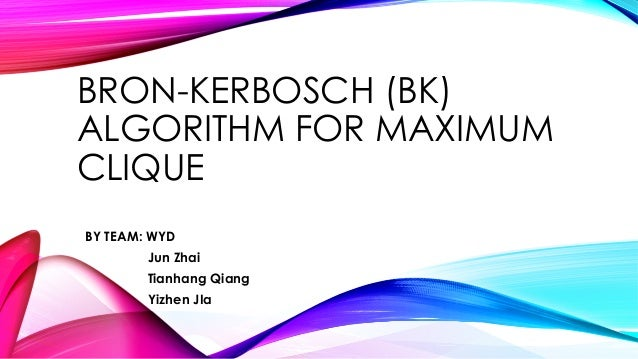Successfully reported this slideshow.
We use your LinkedIn profile and activity data to personalize ads and to show you more relevant ads. You can change your ad preferences anytime.Upcoming SlideShare
Loading in …5
×

# Bron Kerbosch Algorithm - Presentation by Jun Zhai, Tianhang Qiang and Yizhen Jia

2,262 views

Published on

Bron Kerbosch Algorithm - Presentation by Jun Zhai, Tianhang Qiang and Yizhen Jia, for CS 6212 Project P4 at the GWU, Fall 2015 (Prof. Arora's class)

Published in: Education
• Full Name
Comment goes here.

Are you sure you want to Yes No
Your message goes here• Be the first to comment

• Be the first to like this

### Bron Kerbosch Algorithm - Presentation by Jun Zhai, Tianhang Qiang and Yizhen Jia

1. 1. BRON-KERBOSCH (BK) ALGORITHM FOR MAXIMUM CLIQUE BY TEAM: WYD Jun Zhai Tianhang Qiang Yizhen JIa
2. 2. PROBLEM DEFINITION •  Clique: A Clique in an undirected graph G = (V,E) is a subset of the vertex set C ⊆ V, such that for every two vertices in C, there exists an edge connecting the two. •  Maximum Clique: A Clique of the largest possible size in a given graph. •  Maximal Clique: A Clique that cannot be extended by including one more adjacent vertex.
3. 3. WHY IS THIS PROBLEM IMPORTANT? •  Social Network •  BioInformation •  Computing Chemistry
4. 4. HARDNESS OF PROBLEM •  No algorithm can prove a given clique is maximum in polynomial time. •  CSAT problem can be reduced to maximum clique problem in polynomial time •  NP-HARD problem
5. 5. BronKerbosch1(R, P, X): if P and X are both empty: report R as a maximal clique for each vertex v in P: BronKerbosch1(R ⋃ {v}, P ⋂ N(v), X ⋂ N(v)) P := P {v} X := X ⋃ {v} BASIC BK ALGORITHM OUTLINE R: set of vertices that construct the Maximal Clique P: set of possible vertices may be selected X: set of vertices can not construct Maximal Clique
6. 6. BASIC BK ALGORITHM OUTLINE
7. 7. BASIC BK ALGORITHM OUTLINE
8. 8. BronKerbosch1(R, P, X): if P and X are both empty: report R as a maximal clique choose a pivot vertex u in P ⋃ X for each vertex v in P N(u): BronKerbosch1(R ⋃ {v}, P ⋂ N(v), X ⋂ N(v)) P := P {v} X := X ⋃ {v} BK ALGORITHM WITH PIVOT u: pivot vertex in P N(u): neighbor vertices of u
9. 9. BK ALGORITHM WITH PIVOT
10. 10. TIME COMPLEXITY •  Any n-vertex graph has at most 3n/3 Maximal Cliques. •  The worst-case running time of the Bron-Kerbosch algorithm (with a pivot strategy that minimizes the number of recursive calls made at each step) is O(3n/3)
11. 11. UPPER AND LOWER BOUND •  The upper bound is when the graph had the most maximal cliques. So the upper bound should be c*3n/3. •  The lower bound is when the graph is a maximal clique. So the lower bound should be c.
12. 12. REFERENCES https://en.wikipedia.org/wiki/Bron%E2%80%93Kerbosch_algorithm https://tuongphuongtn.files.wordpress.com/2012/11/baocaoseminar2012.pdf https://snap.stanford.edu/class/cs224w-readings/tomita06cliques.pdf https://www.youtube.com/watch?v=132XR-RLNoY